#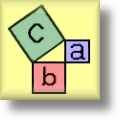Pythagorean Theorem Worksheets

## Working with the Pythagorean Theorem

Here is a graphic preview for all of the Pythagorean Theorem Worksheets. You can select different variables to customize these Pythagorean Theorem Worksheets for your needs. The Pythagorean Theorem Worksheets are randomly created and will never repeat so you have an endless supply of quality Pythagorean Theorem Worksheets to use in the classroom or at home. Our Pythagorean Theorem Worksheets are free to download, easy to use, and very flexible.

These Pythagorean Theorem Worksheets are a great resource for children in 6th Grade, 7th Grade, and 8th Grade.

Click here for a Detailed Description of all the Pythagorean Theorem Worksheets.

## Quick Link for All Pythagorean Theorem Worksheets

Click the image to be taken to that Pythagorean Theorem Worksheet.

##### Pythagorean TheoremPractice ProblemsWorksheets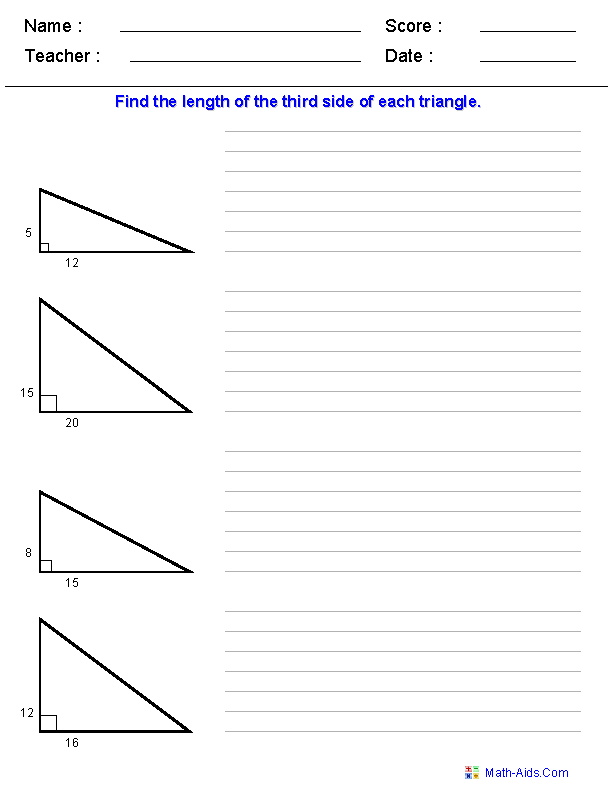##### Distance FormulaSingle Quadrant ProblemsWorksheets##### Distance FormulaFour Quadrant ProblemsWorksheets##### Pythagorean TheoremDefinitions WorksheetsPage 1##### Pythagorean TheoremDefinitions WorksheetsPage 2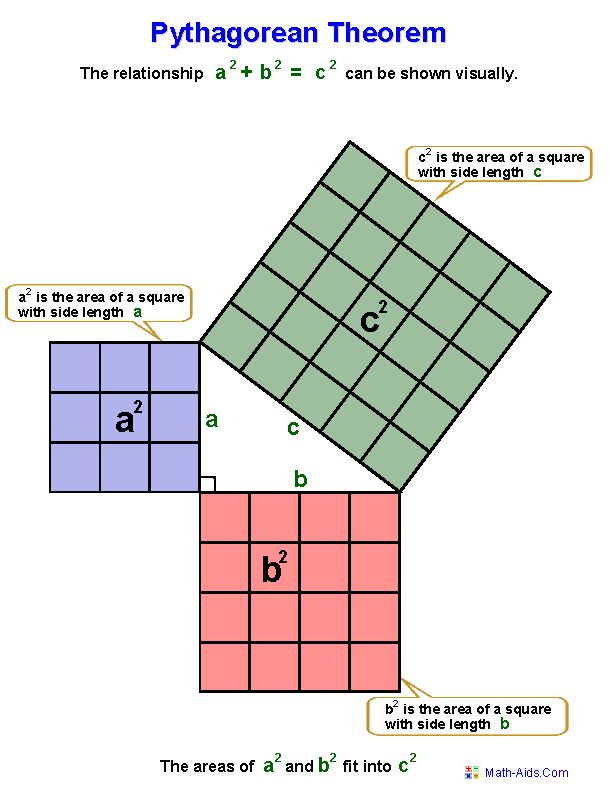##### Pythagorean TheoremDefinitions WorksheetsPage 3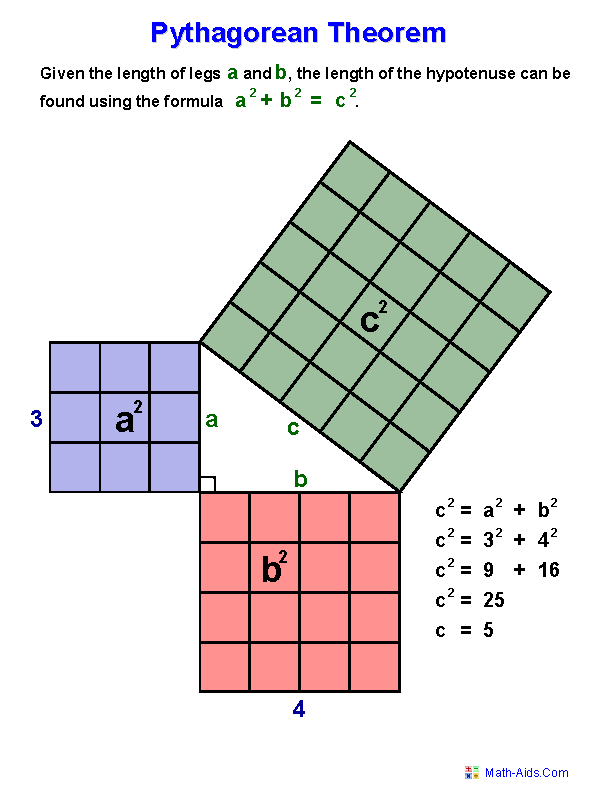##### Pythagorean TheoremDefinitions WorksheetsPage 4##### Pythagorean TheoremDefinitions WorksheetsPage 5##### Pythagorean TheoremDefinitions WorksheetsPage 6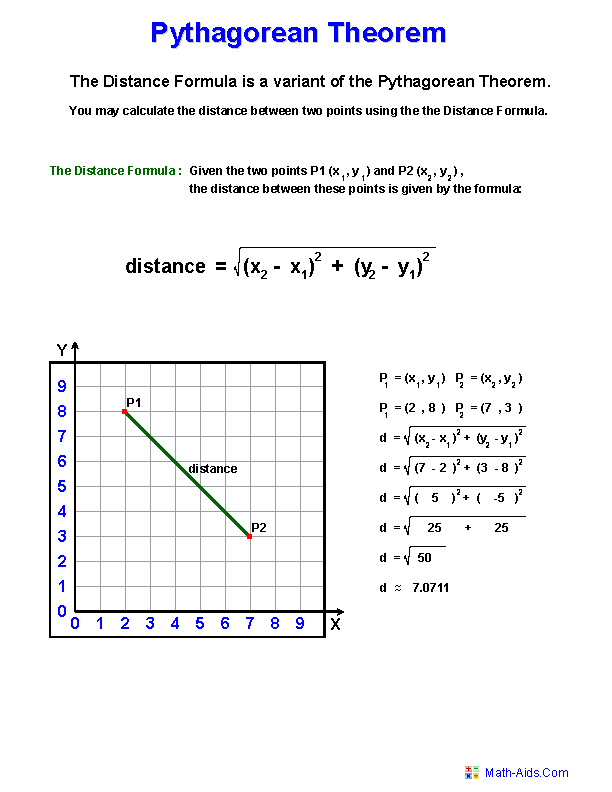Recommended Videos

## Detailed Description for All Pythagorean Theorem Worksheets

Pythagorean Theorem Practice Problems Worksheets
This Pythagorean Theorem Problems Worksheet will produce problems for practicing solving the lengths of right triangles. You may choose the type of numbers and the sides of the triangle. This worksheet is a great resources for the 6th Grade, 7th Grade, and 8th Grade.

Distance Formula Problems Worksheets
This Pythagorean Theorem Distance Problems Worksheet will produce problems for practicing solving distances between two sets of points on a coordinate plane. You may choose between single quadrant or four quadrant problems. This worksheet is a great resources for the 6th Grade, 7th Grade, and 8th Grade.

Pythagorean Theorem Definitions and Examples Worksheets
These Pythagorean Theorem Worksheets will produce colorful and visual pages that contain definitions and examples for the Pythagorean Theorem and the Distance Formula. These worksheets are great resources for the 6th Grade, 7th Grade, and 8th Grade.